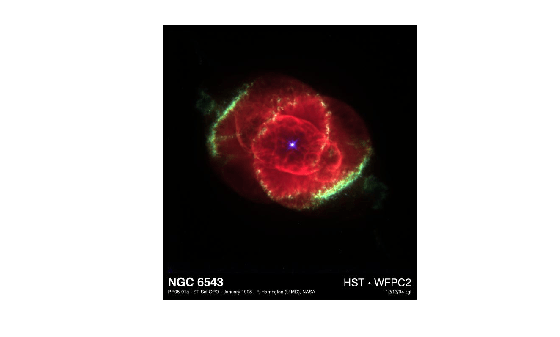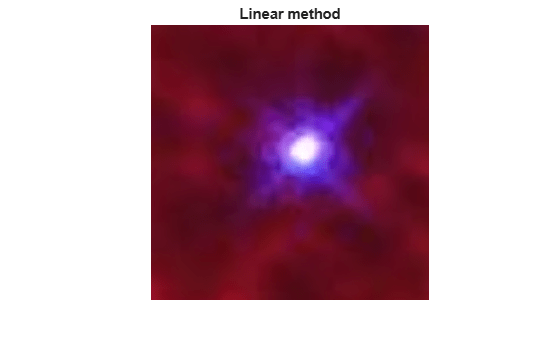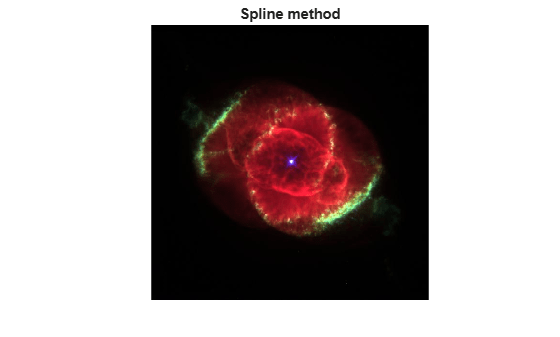# Resample Image with Gridded Interpolation

This example shows how to use `griddedInterpolant` to resample the pixels in an image. Resampling an image is useful for adjusting the resolution and size, and you also can use it to smooth out the pixels after zooming.

Load and show the image `ngc6543a.jpg`, which is a Hubble Space Telescope image of the planetary nebulae NGC 6543. This image displays several interesting structures, such as concentric gas shells, jets of high-speed gas, and unusual knots of gas. The matrix `A` that represents the image is a 650-by-600-by-3 matrix of `uint8` integers.

```A = imread('ngc6543a.jpg'); imshow(A)```### Create Interpolant

Create a gridded interpolant object for the image. `griddedInterpolant` only works for double-precision and single-precision matrices, so convert the `uint8` matrix to `double`. To interpolate each RGB channel of the image, specify two grid vectors to describe the sample points in the first two dimensions. The grid vectors are grouped together as column vectors in a cell array `{xg1,xg2,...,xgN}`. With this formulation, `griddedInterpolant` treats the 3-D matrix as containing multiple 2-D data sets defined on the same grid.

```sz = size(A); xg = 1:sz(1); yg = 1:sz(2); F = griddedInterpolant({xg,yg},double(A));```

### Resample Image Pixels

Use the sizes of the first two matrix dimensions to resample the image so that it is 120% the size. That is, for each 5 pixels in the original image, the interpolated image has 6 pixels. Evaluate the interpolant at the query points with the syntax `F({xq,yq})`. `griddedInterpolant` evaluates each page in the 3-D image at the query points.

```xq = (0:5/6:sz(1))'; yq = (0:5/6:sz(2))'; vq = uint8(F({xq,yq})); imshow(vq) title('Higher Resolution')```Similarly, reduce the size of the image by querying the interpolant with 55% fewer points than the original image. While you can simply index into the original image matrix to produce lower resolution images, interpolation enables you to resample the image at noninteger pixel locations.

```xq = (0:1.55:sz(1))'; yq = (0:1.55:sz(2))'; vq = uint8(F({xq,yq})); figure imshow(vq) title('Lower Resolution')```### Smooth Out Zooming Artifacts

As you zoom in on an image, the pixels in the region of interest become larger and detail in the image is quickly lost. You can use image resampling to smooth out these zooming artifacts.

Zoom in on the bright spot in the center of the original image. (The indexing into `A` is to center this bright spot in the image so that subsequent zooming does not push it out of the frame.)

```imshow(A(1:570,10:600,:),'InitialMagnification','fit') zoom(10) title('Original Image, 10x Zoom')```Query the interpolant `F` to reproduce this zoomed image (approximately) with 10x higher resolution. Compare the results from several different interpolation methods.

```xq = (1:0.1:sz(1))'; yq = (1:0.1:sz(2))'; F.Method = 'linear'; vq = uint8(F({xq,yq})); imshow(vq(1:5700,150:5900,:),'InitialMagnification','fit') zoom(10) title('Linear method')``````F.Method = 'cubic'; vq = uint8(F({xq,yq})); imshow(vq(1:5700,150:5900,:),'InitialMagnification','fit') zoom(10) title('Cubic method')``````F.Method = 'spline'; vq = uint8(F({xq,yq})); imshow(vq(1:5700,150:5900,:),'InitialMagnification','fit') zoom(10) title('Spline method')```